# For the beam shown, EI is constant. (Figure 1) Part A Determine the horizontal reaction at...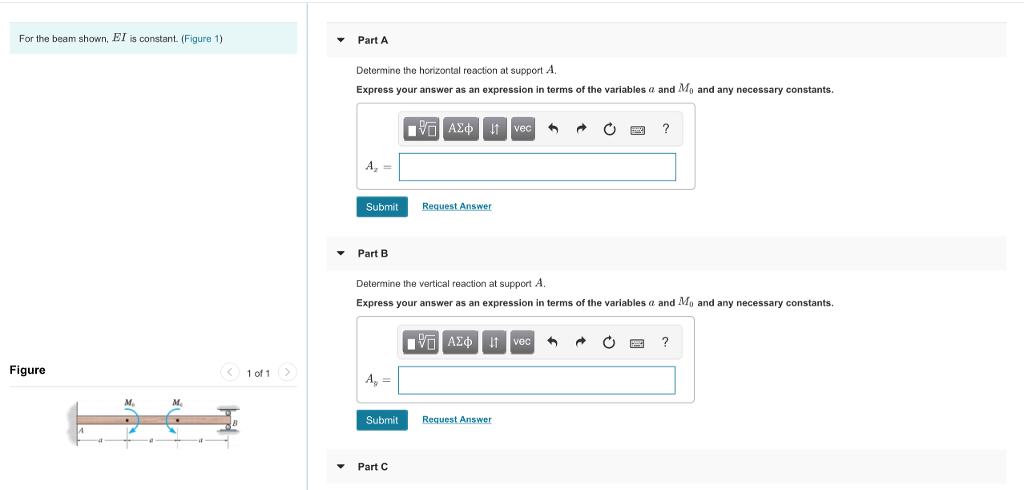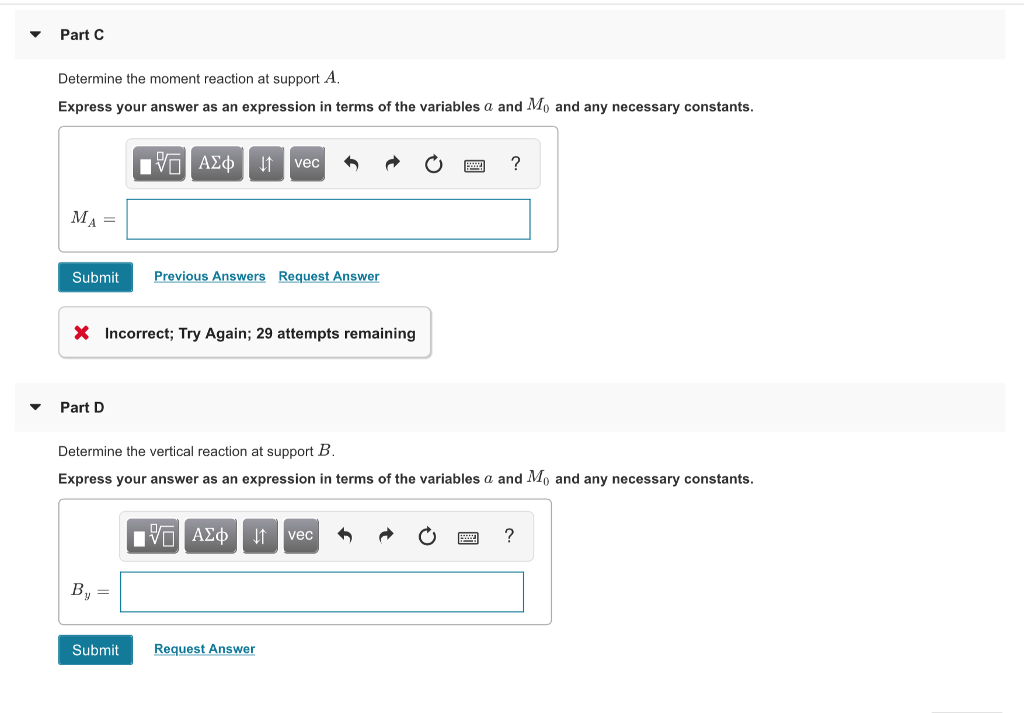For the beam shown, EI is constant. (Figure 1) Part A Determine the horizontal reaction at support A. Express your answer as an expression in terms of the variables a and Mo and any necessary constants. Submit Request Answer Part B Determine the vertical reaction at support A Express your answer as an expression in terms of the variables a and Mo and any necessary constants. ΑΣφ Figure < 1011 Submit Request Answer Part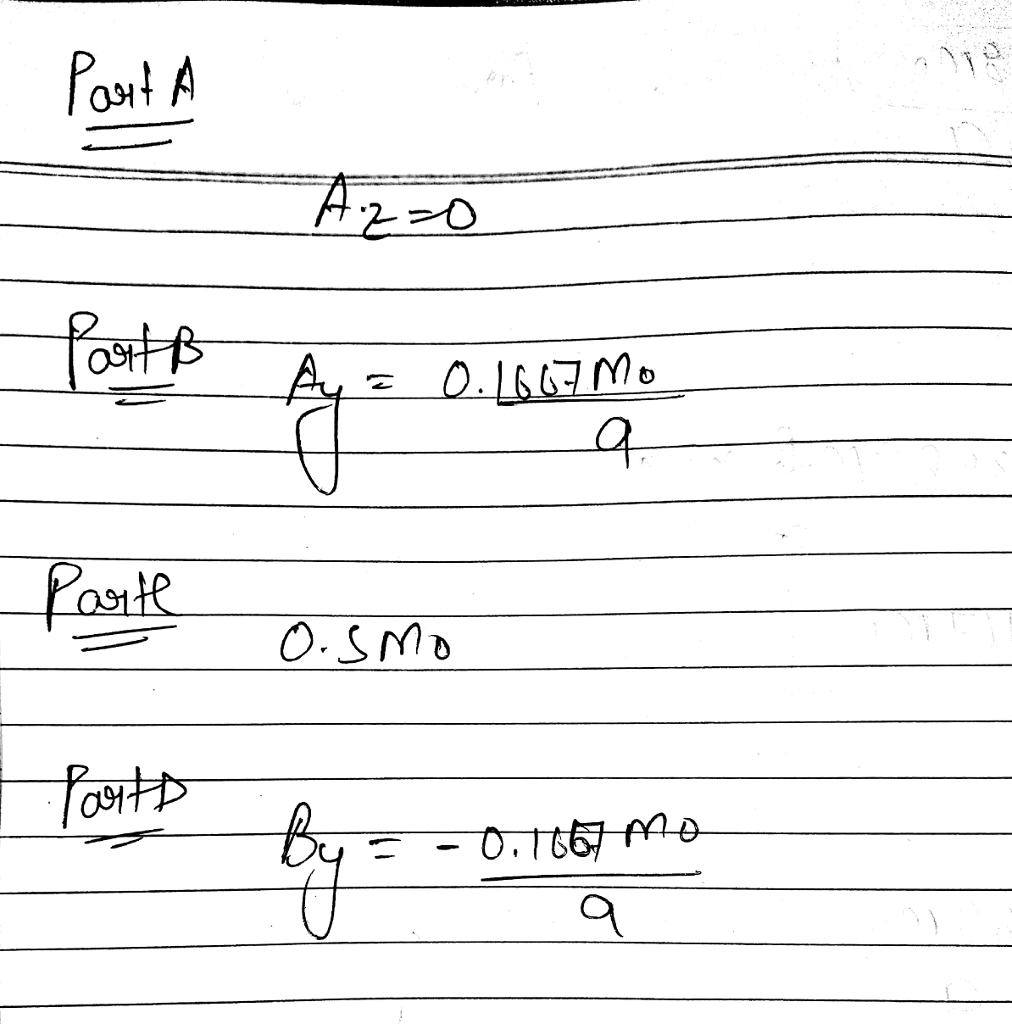##### Add Answer to: For the beam shown, EI is constant. (Figure 1) Part A Determine the horizontal reaction at...
Similar Homework Help Questions
• ### <HW#9 (Chapter 12) Problem 12.120 For the beam shown, I E is constant. (Figure 1) P...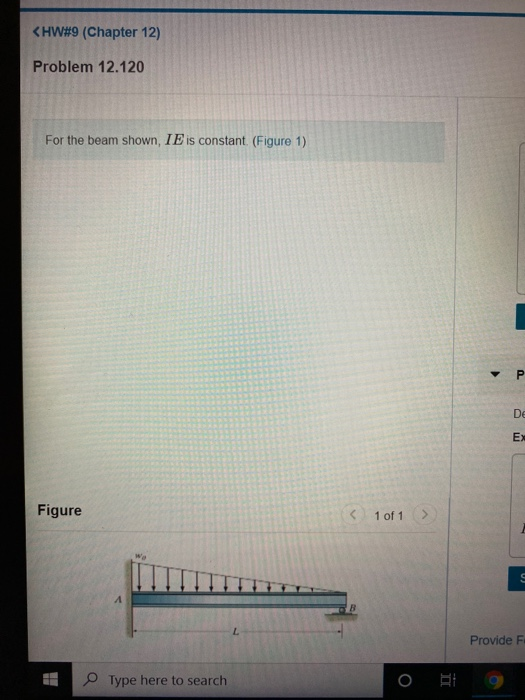<HW#9 (Chapter 12) Problem 12.120 For the beam shown, I E is constant. (Figure 1) P De EX Figure < 1 of 1 > Provide F Type here to search Part B Determine the vertical reaction at support A. Express your answer as an expression in terms of the variables wo and L and any necessary constants. VAXO 11 vec o ? a р y 8 € n e K 2 M V р o T ф X y 0...

• ### Part A Determine the deflection of end B of the cantilever beam. EI is constant. (Figure...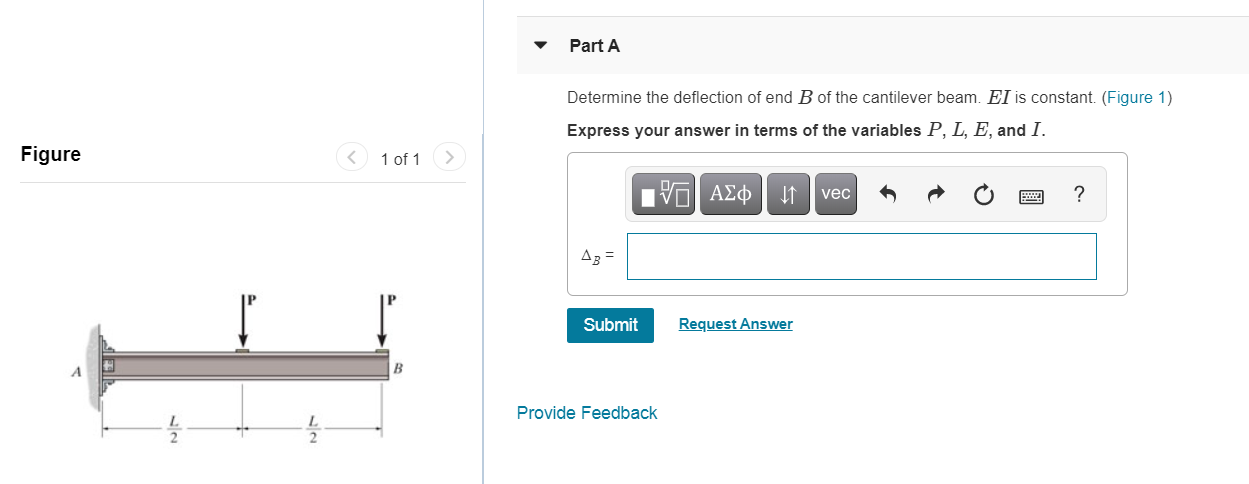Part A Determine the deflection of end B of the cantilever beam. EI is constant. (Figure 1) Express your answer in terms of the variables P, L, E, and I. Figure < 1 of 1 ΡΕΙ ΑΣφ JT vec ? A3 = Submit Request Answer | В Provide Feedback

• ### Consider the beam shown in (Figure 1). EI is constant. Part A Determine the x component...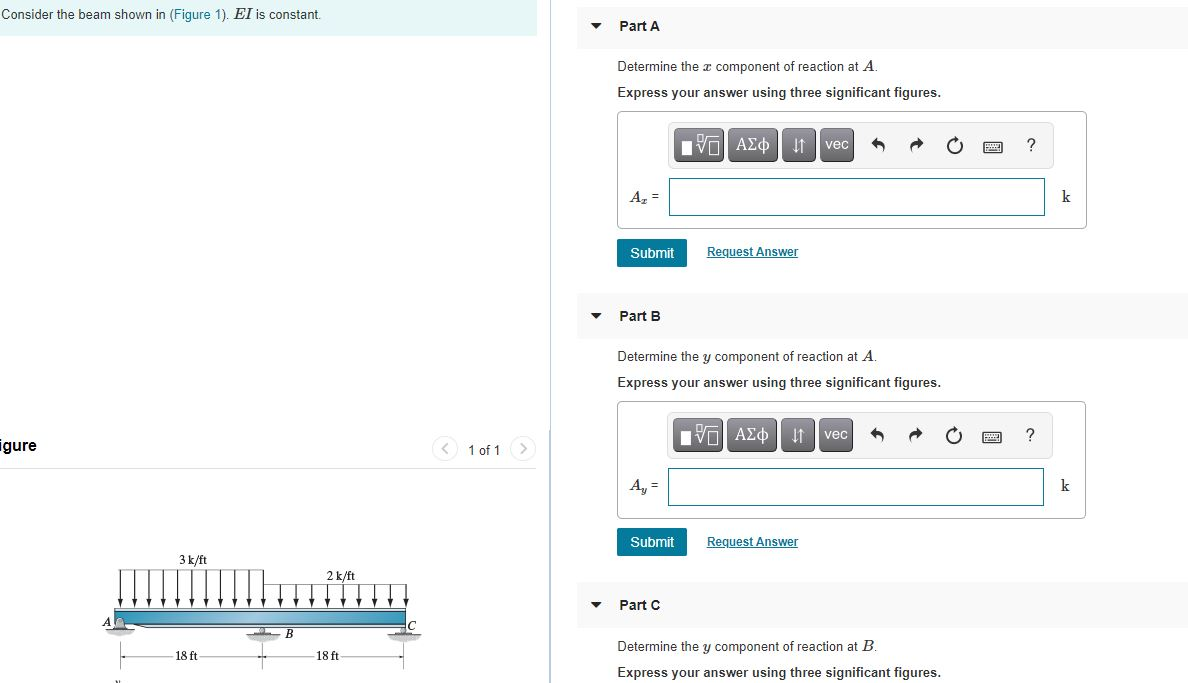Consider the beam shown in (Figure 1). EI is constant. Part A Determine the x component of reaction at A. Express your answer using three significant figures. ΟΙ ΑΣΦ | It vec ? Az = k Submit Request Answer Part B Determine the y component of reaction at A. Express your answer using three significant figures. IVO AXO 11 vec ? igure < 1 of 1 Submit Request Answer 3 k/ft 2 k/ft Part C 18 ft 18 ft Determine...

• ### Problem 12.17 (Fiet) Part A Determine the equations of the elastic curve for the beam using...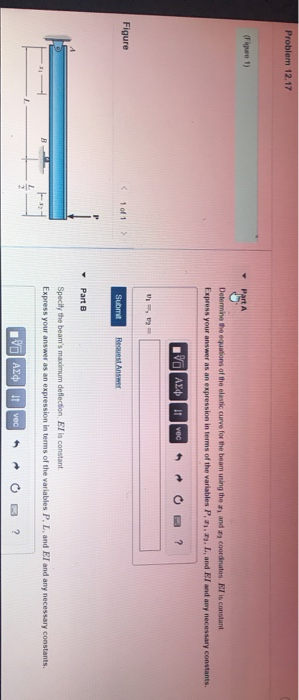Problem 12.17 (Fiet) Part A Determine the equations of the elastic curve for the beam using them and my coordinates 7 is constant Express your answer as an expression in terms of the variables P. 2.). L, and El and any necessary constants. 190 AED vec ? , Figure 1 of 1 Submit Request Answer Part 8 Specify the beam's maximum deflection El is constant Express your answer as an expression in terms of the variables P. L and El...

• ### Part A Consider the beam shown in (Figure 1). EI is constant Determine the equation of the elastic curve tor the beam using the z1 coordinate tor 0 L/2 Express your answer in terms of the variables P...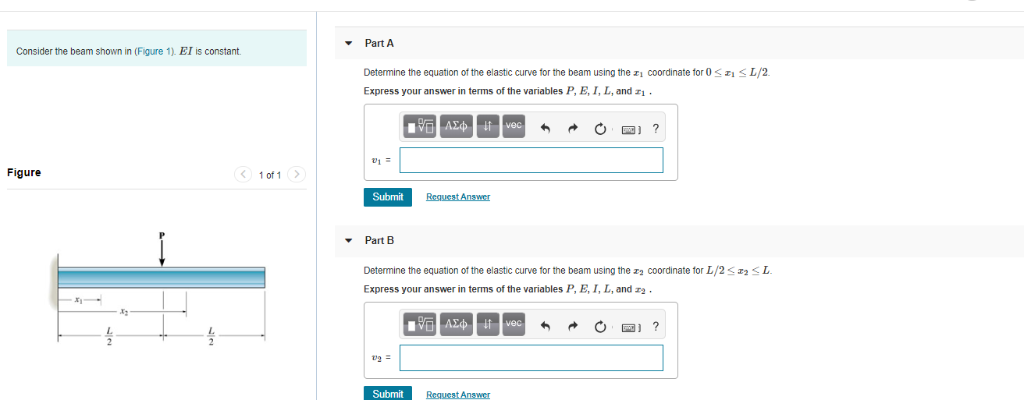Part A Consider the beam shown in (Figure 1). EI is constant Determine the equation of the elastic curve tor the beam using the z1 coordinate tor 0 L/2 Express your answer in terms of the variables P, E, I, L, and 01F Figure 1 of 1 Submit Part B Determine the equation of the elastic curve tor the beam using the 2 coordinate for L/2L Express your answer in terms of the variables P, E, I, L, and z...

• ### Part A The beam is subjected to the linearly varying distributed load Determine the maximum deflection of the beam. EI...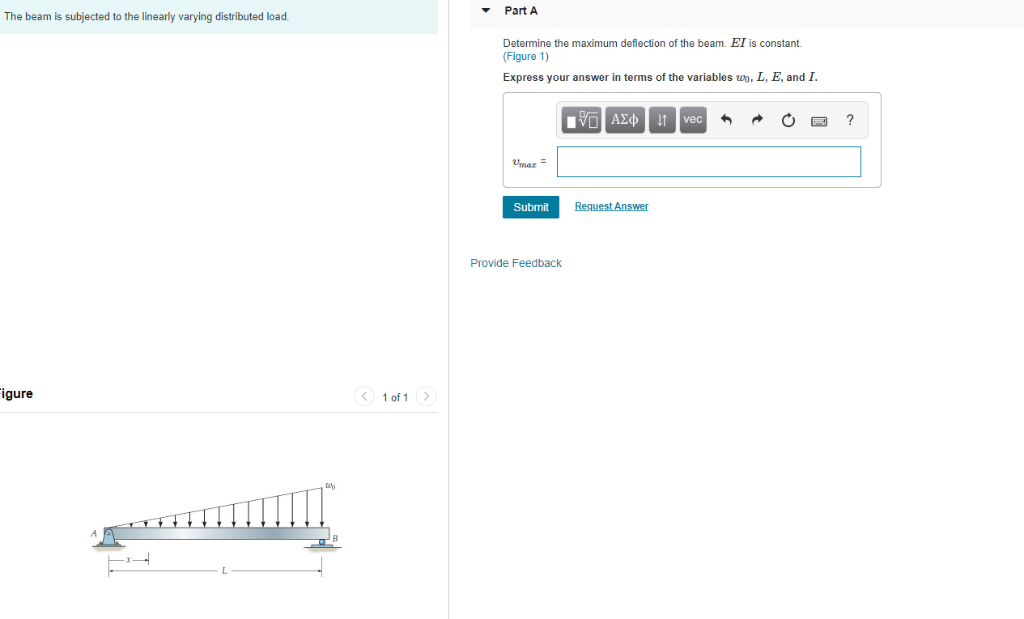Part A The beam is subjected to the linearly varying distributed load Determine the maximum deflection of the beam. EI is constant. (Figure 1) Express your answer in terms of the variables wp, L, E, and I Unaz- Submit Provide Feedback igure 1 of 1> Part A The beam is subjected to the linearly varying distributed load Determine the maximum deflection of the beam. EI is constant. (Figure 1) Express your answer in terms of the variables wp, L, E,...

• ### Problem 9.17 Consider the frame shown in (Figure 1). EI is constant. Part A Determine the...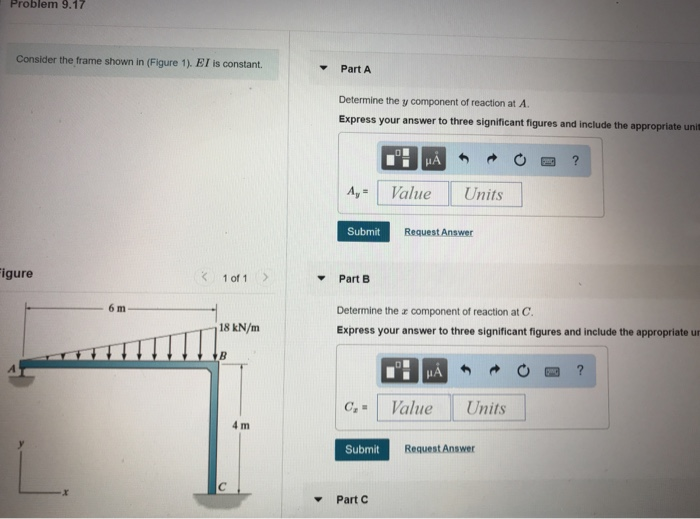Problem 9.17 Consider the frame shown in (Figure 1). EI is constant. Part A Determine the y component of reaction at A. Express your answer to three significant figures and include the appropriate unit A- Value Units Submit Request Answer igure 1 of 1 Part B 6 m Determine the z component of reaction at C. Express your answer to three significant figures and include the appropriate u 18 kN/m G-1 Value Units 4 m Submit Request Answer Part C

• ### so if you sure from what are u doing Review Part A Consider the beam shown...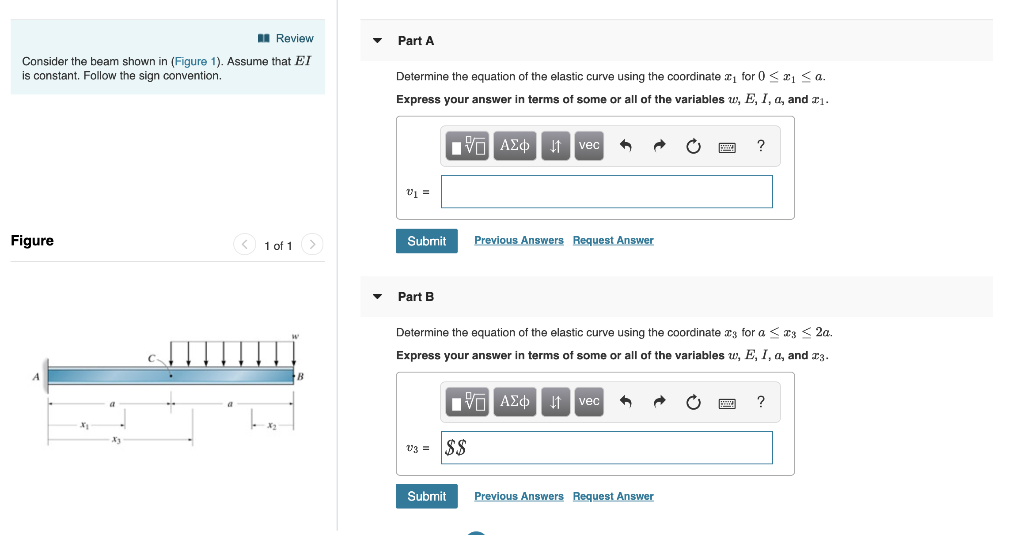so if you sure from what are u doing Review Part A Consider the beam shown is constant. Follow the sign convention. (Figure 1). Assume that EI Determine the equation of the elastic curve using the coordinate for 0 1 < a. Express your answer in terms of some or all of the variables u, E, I, a, and . VAEd vec Figure Submit Previous Answers Request Answer 1 of 1 Part B Determine the equation of the elastic curve...

• ### Consider the beam shown in (Figure 1). EI is constant. Assume B and C are rollers...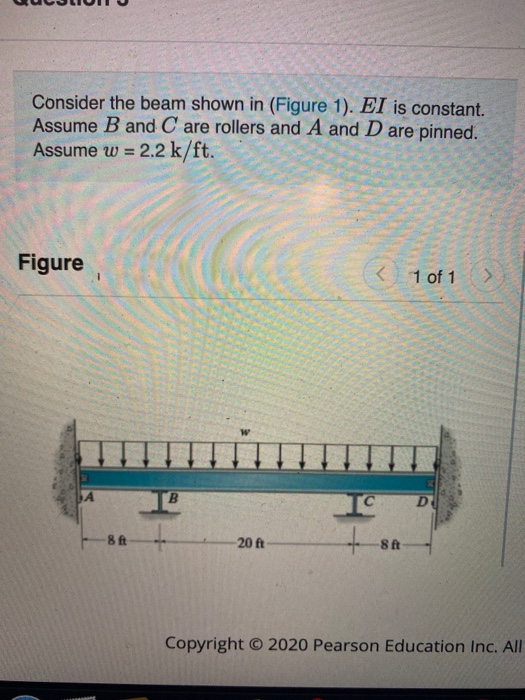Consider the beam shown in (Figure 1). EI is constant. Assume B and C are rollers and A and D are pinned. Assume w = 2.2 k/ft. Figure < - 1 of 1 > IA D 8 ft -20 ft 8 ft Copyright © 2020 Pearson Education Inc. All 3 of 3 Part A Determine the moment MBA acting on span AB at B measured clockwise. Express your answer using three significant figures. Enter positive value if the moment is...

• ### Consider the frame shown in (Figure 1). EI is constant. Part A Determine the y component...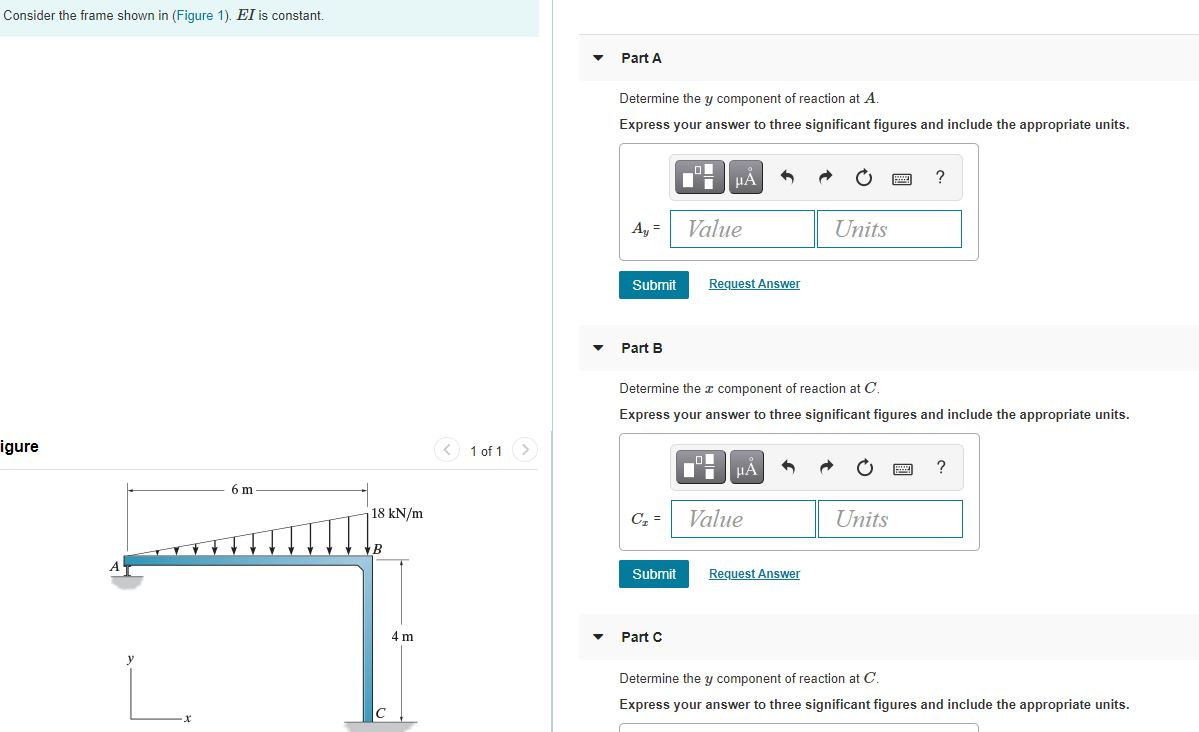Consider the frame shown in (Figure 1). EI is constant. Part A Determine the y component of reaction at A. Express your answer to three significant figures and include the appropriate units. -0% Ti A ? Ay = Value Units Submit Request Answer Part B Determine the component of reaction at C. Express your answer to three significant figures and include the appropriate units. igure 1 of 1 НА ? 6 m 18 kN/m C = Value Units B Submit...

Free Homework App